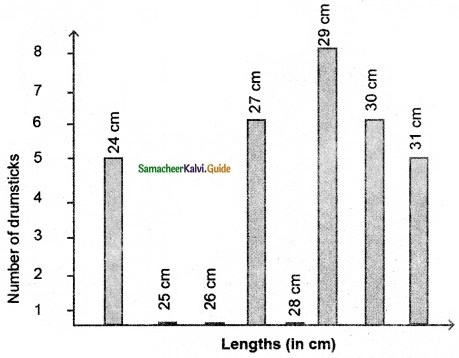Students can download Maths Chapter 5 Statistics Ex 5.4 Questions and Answers, Notes, Samacheer Kalvi 6th Maths Guide Pdf helps you to revise the complete Tamilnadu State Board New Syllabus, helps students complete homework assignments and to score high marks in board exams.

Tamilnadu Samacheer Kalvi 6th Maths Solutions Term 1 Chapter 5 Statistics Ex 5.4

Miscellaneous Practice Problems

Question 1.
The heights (in centimeters) of 40 children are.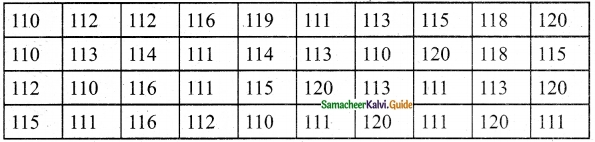Prepare a tally marks table.
Solution:Question 2.
There are 1000 students in a school. Data regarding the mode of transport of the students is given below. Draw a pictograph to represent the data.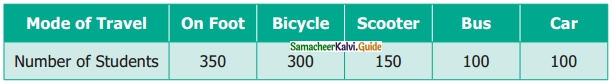Solution:
Mode of transport of the students
Scale: 1 Unit=100 StudentsQuestion 3.
The following pictograph shows the total savings of a group of friends in a year. Each .picture represents a saving of Rs.100. Answer the following questions.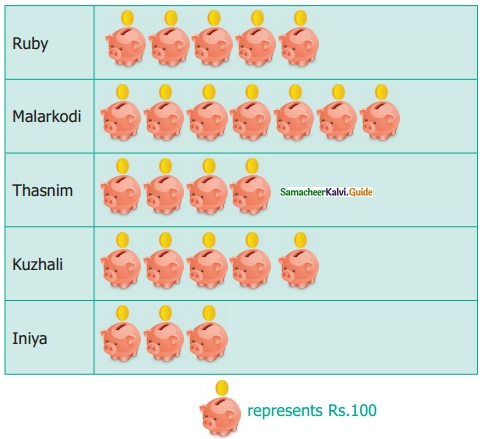(i) What is the Ratio of Ruby’s saving to that of Thasnim’s?
(ii) What is the ratio of Kuzhali’s savings to that of others?
(iii) How much is Iniya’s savings?
(iv) Find the total amount of savings of all the friends?
(v) Ruby and Kuzhali save the same amount. Say True or False.
Solution:
(i) Ratio of Ruby’s saving to that of Thasnim’s
$$=\frac{\text { Ruby’s saving }}{\text { Thasnim’s saving }}=\frac{5 \times 100}{4 \times 100}=\frac{5}{4}=5: 4$$
Ratio of Ruby’s saving to that of Thasnims = 5 : 4
(ii) Ratio of Kuzhali’s savings to that of others
$$=\frac{\text { kuzhali’s saving }}{\text { others saving }}=\frac{5 \times 100}{19 \times 100}=\frac{5}{19}=5: 19$$
Ratio of Kuzhali’s saving to that of others = 5 : 19
(iii) Iniya’s saving = 3 × 100 = ₹ 300
(iv) Saving of all the friends = (5 + 7 + 4 + 5 + 3) × 100 = 24 × 100 = ₹ 2400.
Total savings = ₹ 2400
(v) True.Challenging Problems

Question 4.
The table shows the numbers of moons that orbit each of the planets in oar solar system.Make a Bar graph for the above data.
Solution:
Number of moons that orbit each of the planets in our solar system
Scale: 1 Unit = 2 moonsQuestion 5.
The prediction of the weather in the month of September is given below.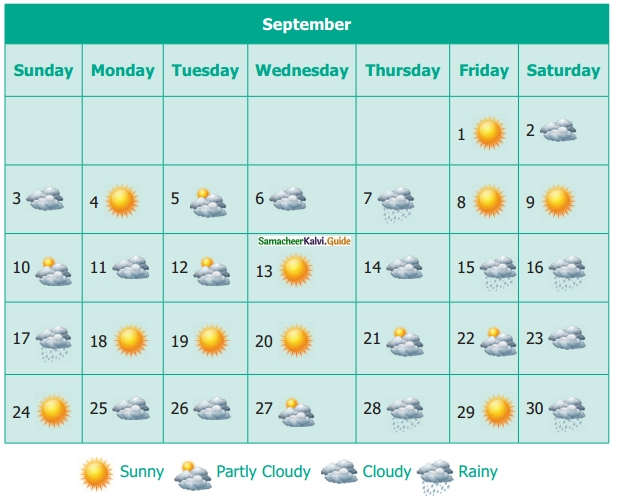(i) Make a frequency table of the types of weather by reading the calendar.
(ii) How many days are either cloudy or partly cloudy?
(iii) How many days do not have rain? Give two ways to find the answer?
(iv) Find the ratio of the number of Sunny days to Rainy days.
Solution:
(i)(ii) 14 days
(iii) 24 days (30 – 6 = 24 days)
(iv) 10 : 6Question 6.
26 students were interviewed to find out what they want they to become in future. Their responses are given in the following table.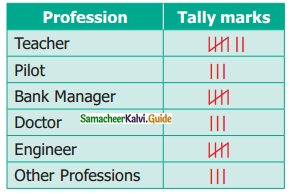Represent this data using pictograph.
Solution:
Students responses in an interview about their future profession
Scale: 1 Unit = 1 student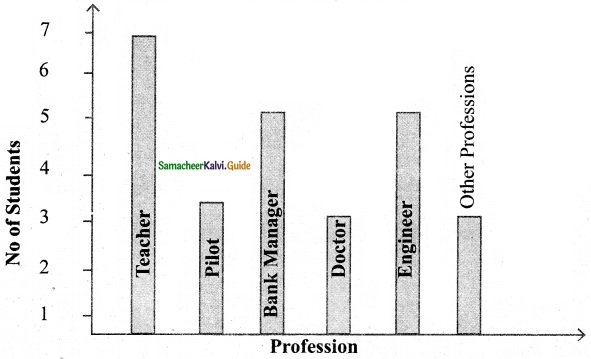Question 7.
Yasmin of class VI was given a task to count the number of books which are biographies, in her school library. The information collected by her is represented as follows.Observe the pictograph and answer the following questions.
(i) Which title has the maximum number of biographies?
(ii) Which title has the minimum number of biographies?
(iii) Which title has exactly half the number of biographies as Novelists?
(iv) How many biographies are there on the title of sportspersons?
(v) What is the total number of biographies in the library?
Solution:
(i) ‘The title Novelists’ have the maximum number of biographies
(ii) ‘The title Scientists’ have a minimum number of biographies.
(iii) ‘Sportspersons’ title has exactly half the number of biographies as Novelist.
(iv) $$(1 \times 20)+\frac{20}{4}=20+5=25$$ biographies are there in the title sportsperson.
(v) 8 × 20 = 160 biographies are there in the library.Question 8.
The bar graph illustrates the results of a survey conducted on vehicles crossing over a Toll Plaza in one hour.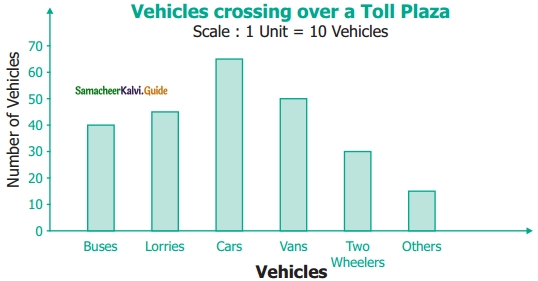Observe the bar graph carefully and fill up the following table.Solution:
Vans = 50; Buses = 40; Cars = 65; Others = 15
Total Vehicles = 245Question 9.
The lengths (in the nearest centimeter) of 30 drumsticks are given as follows.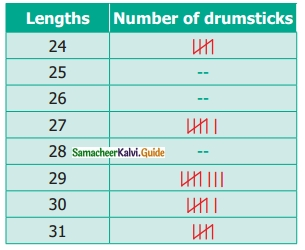Draw the bar graph showing the same information.
Solution:
The lengths (in nearest cm) of drumsticks
Scale : 1 Unit = 1 drumstick